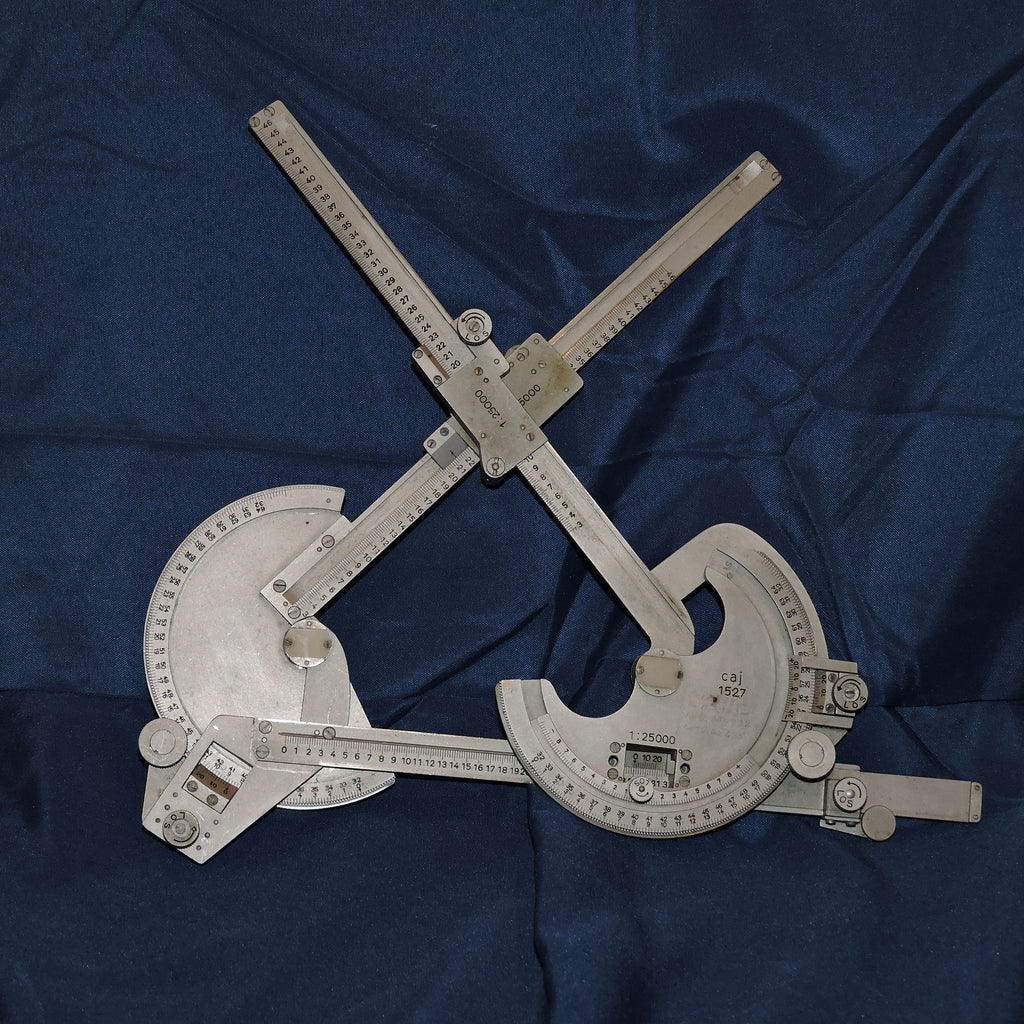Preserving Warbird History...one artifact at a time.# Measuring Triangle (Meßdreieck) for German MG 34 and MG 42 Machine Guns

\$295.00

Here is a highly unusual and complicated instrument... a German Meßdreieck (Messdreieck), or measuring triangle, used by MG 34 or MG 42 Machine Gun crews during WWII. The measuring triangle was used to optimize the fire efficiency of machine guns and to calculate distances.  It may also have been used to support artillery aiming.

We found a chatroom with the following information from "Roger":

It resolves trig functions, but cannot directly calculate where a projectile will land because it has no input for velocity or ballistic coefficient. I have no idea why, but this thing divides a circle into 64 units, or 5.625 degrees per unit. I will give a simple example of it's use, but this will work for any triangle. For this example I will call the short scale between the two angle adjustment knobs "X." With X at the bottom, I will call the long arm on the left "Y" and the arm on the right side "R." Also note that if you set both angles to same number the arms are parallel.

Set the angle scale on the left to 16 (90 degrees) so will have a right triangle. Set the X scale to 10. Set the right side angle to 20 (112.5 degrees.) Here is the secret. Both angles start from the same place, so the angle on the right side is in fact 180 - 112.5 or 67.5 degrees. Next, cos(theta) = X / R or R = 10 / tan(67.5) = 26.1. My R scale reads 26. Next, sin(theta) = Y / R so Y = sin(67.5) x 26.1 = 24.1. My Y scale reads 24.

Just as with any trig function, you need 2 angles and 1 side, or 2 sides and 1 angle to find the other 3 numbers.

This example is made by an unknown manufacturer "caj".  It is undated, but our research leads us to believe that it is 1930-40's vintage. All LOS knobs turn to loosen or tighten the device, and all slides work as they should. When closed, measures ~12 inches x ~5 inches.

A very cool instrument indeed.